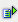The function sign (num) returns the value 1, if the value (num) is positive, the value -1, if the value is negative, and the value 0, if the value=0.

Syntax: =sign(num)Example =sign(2.2) is 1 =sign(-2.2) is -1 =sign(0,0) is 0